# Machine learning questions

Last updated on：a year ago

I also get some review quiz from cousera.

# Intro

Question 1

A computer program is said to learn from experience E with

​ respect to some task T and some performance measure P if its

​ performance on T, as measured by P, improves with experience E.

​ Suppose we feed a learning algorithm a lot of historical weather

​ data, and have it learn to predict weather. In this setting, what is T?

P: The probability of it correctly predicting a future date’s weather.

E: The process of the algorithm examining a large amount of historical weather data.

Question 2

The amount of rain that falls in a day is usually measured in either millimeters (mm) or inches. Suppose you use a learning algorithm to predict how much rain will fall tomorrow. Would you treat this as a classification or a regression problem?

-[x] Regression

Question 4

Some of the problems below are best addressed using a supervised learning algorithm, and the others with an unsupervised learning algorithm.

Which of the following would you apply supervised learning to?

Examine the statistics of two football teams, and predict which team will win tomorrow’s match (given historical data of teams’ wins/losses to learn from).

Given genetic (DNA) data from a person, predict the odds of him/her developing diabetes over the next 10 years.

Which of the following would you apply unsupervised learning to?

Examine a large collection of emails that are known to be spam email, to discover if there are sub-types of spam mail.

Take a collection of 1000 essays written on the US Economy, and find a way to automatically group these essays into a small number of groups of essays that are somehow “similar” or “related”.

# Linear regression with one variable

Question 4

In the given figure, the cost function $J(\theta_0,\theta_1)$has been plotted against $\theta_0$ and $\theta_ 1$, as shown in ‘Plot 2’. The contour plot for the same cost function is given in ‘Plot 1’. Based on the figure, choose the correct options (check all that apply).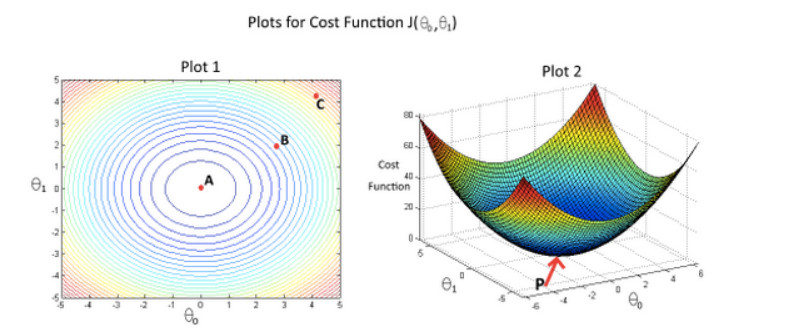If we start from point B, gradient descent with a well-chosen learning rate will eventually help us reach at or near point A, as the value of cost function $J(\theta_0,\theta_1)$ is minimum at A.

Point P (the global minimum of plot 2) corresponds to point A of Plot 1.

Question 5

Suppose that for some linear regression problem (say, predicting housing prices as in the lecture), we have some training set, and for our training set we managed to find some $\theta_0$, $\theta_1$ such that $J(\theta_0, \theta_1)=0$.

Which of the statements below must then be true? (Check all that apply.)

Our training set can be fit perfectly by a straight line, i.e., all of our training examples lie perfectly on some straight line.

Gradient decent may not get stuck at a local minimum.

Question 4

Let f be some function so that

$f(\theta _ 0, \theta _ 1)$ outputs a number. For this problem,

f is some arbitrary/unknown smooth function (not necessarily the

cost function of linear regression, so f may have local optima).

Suppose we use gradient descent to try to minimize $f(\theta _ 0, \theta _ 1)$ as a function of $\theta_0$ and $\theta_1$. Which of the

following statements are true? (Check all that apply.)

If $\theta_0$ and $\theta_1$ are initialized at a local minimum, then one iteration will not change their values.

If the learning rate is too small, then gradient descent may take a very long time to converge.

Depending on the initial condition, gradient descent may end up at different local optima.

# Linear regression with multiple variables

Question 4

Say you have two column vectors v and w, each with 7 elements (i.e., they have dimensions 7x1). Consider the following code:

z = 0;
for i = 1:7
z = z + v(i) * w(i)
end

Which of the following vectorizations correctly compute z? Check all that apply.

• z = v’ * w;
• z = v * w’; (The dimension is 7x7)
• z = sum (v .* w);
• z = v .* w;

Question 5

​ Which of the following are reasons for using feature scaling?

It speeds up gradient descent by making it require fewer iterations to get to a good solution.

# Logistic regression

Question 2

Suppose you have the following training set, and fit a logistic regression classifier $h_\theta(x) = g(\theta_0 + \theta_1x_1 + \theta_2 x_2)$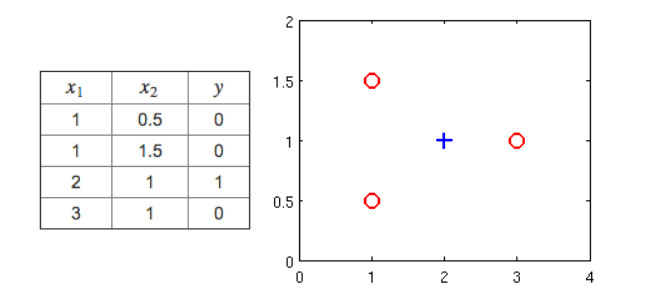Which of the following are true? Check all that apply.

$J(\theta)$ will be a convex function, so gradient descent should converge to the global minimum.

Adding polynomial features (e.g., instead using $h_\theta(x) = g(\theta_0 + \theta_1x_1 + \theta_2 x_2 + \theta_3 x_1^2 + \theta_4 x_1 x_2 + \theta_5 x_2^2)$ could increase how well we can fit the training data.

The positive and negative examples cannot be separated using a straight line. So, gradient descent will fail to converge.

Because the positive and negative examples cannot be separated using a straight line, linear regression will perform as well as logistic regression on this data.

Question 4

​ Which of the following statements are true? Check all that apply.

The one-vs-all technique allows you to use logistic regression for problems in which each $y^{(i)}$ comes from a fixed, discrete set of values.

For logistic regression, sometimes gradient descent will converge to a local minimum (and fail to find the global minimum). This is the reason we prefer more advanced optimization algorithms such as fminunc (conjugate gradient/BFGS/L-BFGS/etc).

Since we train one classifier when there are two classes, we train two classifiers when there are three classes (and we do one-vs-all classification).

The cost function $J(\theta)$ for logistic regression trained with $m \geq 1$ examples is always greater than or equal to zero.

# Learning

Question1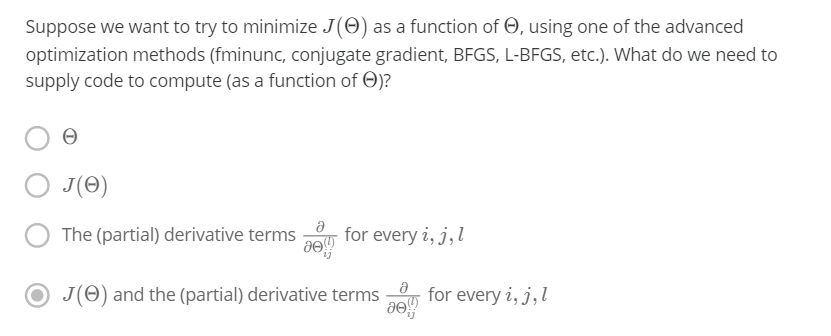Question2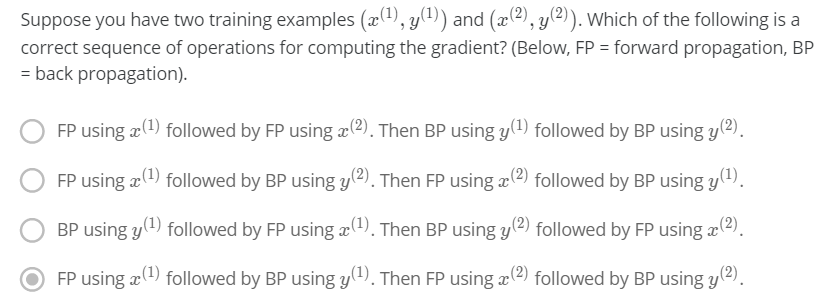Question3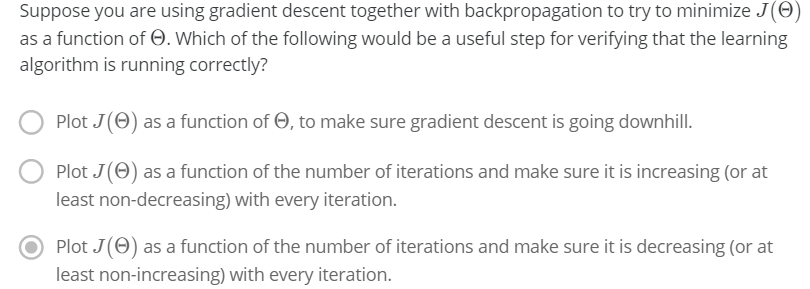Question4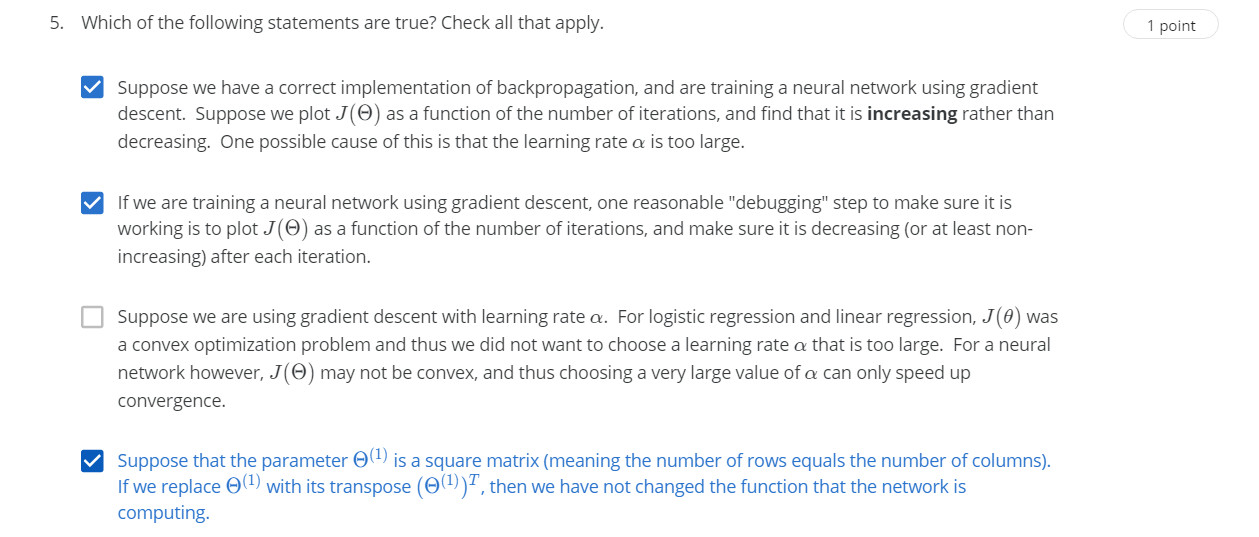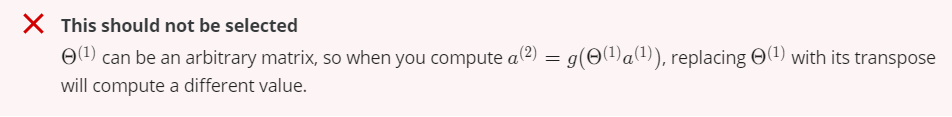Question5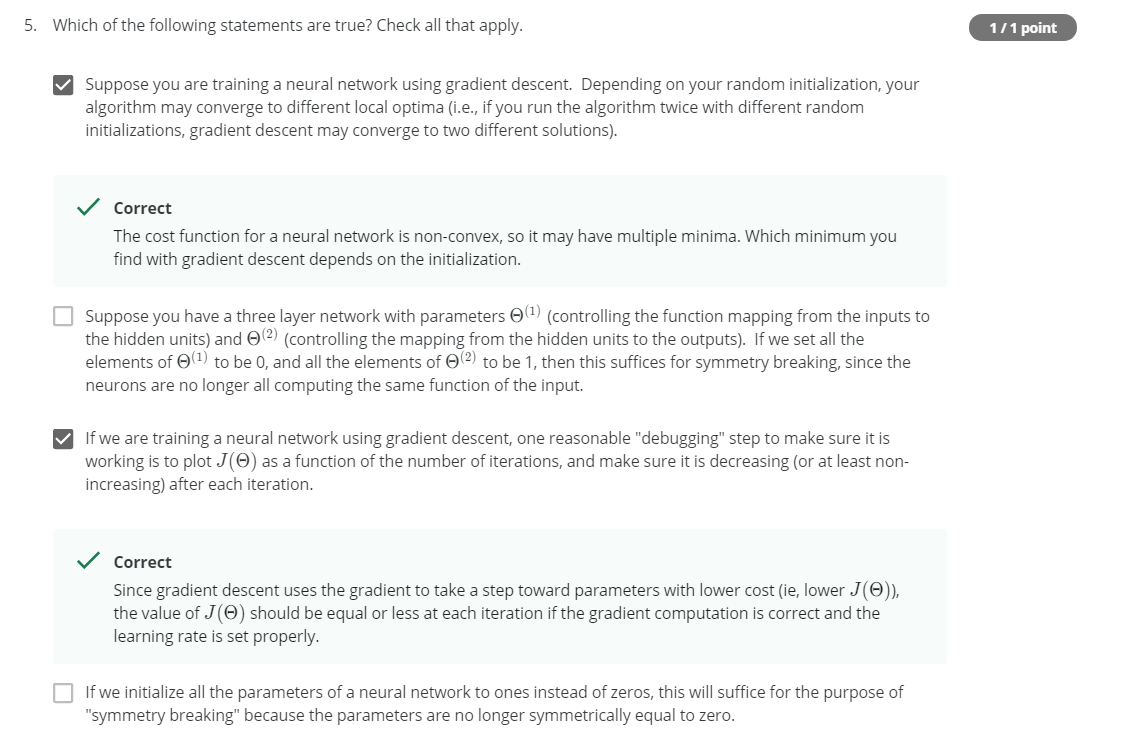A diagnostic can sometimes rule out certain courses of action (changes to your learning algorithm) as being unlikely to improve its performance significantly.

Question1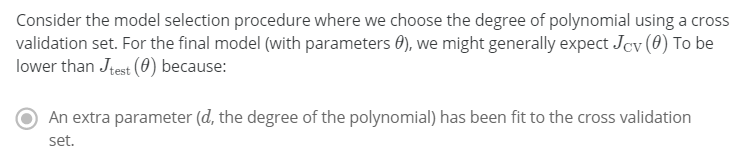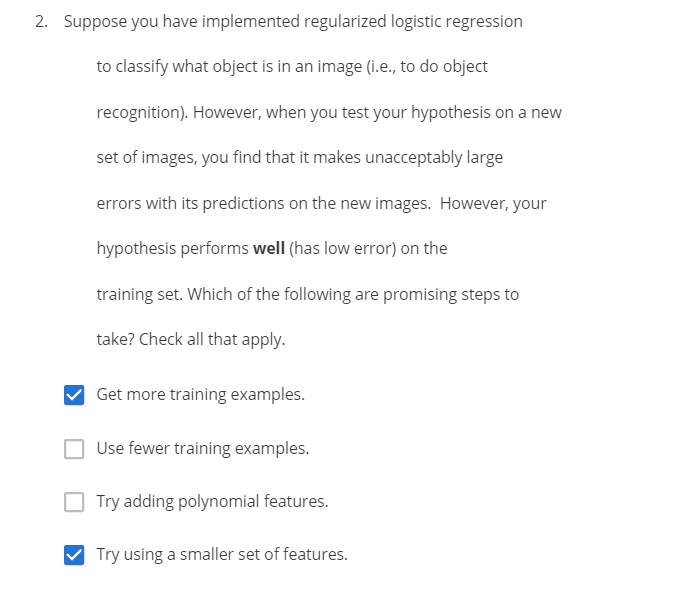Question2: Which of the following statements are true? Check all that apply.

• Suppose you are training a logistic regression classifier using polynomial features and want to select what degree polynomial (denoted in the lecture videos) to use. After training the classifier on the entire training set, you decide to use a subset of the training examples as a validation set. This will work just as well as having a validation set that is separate (disjoint) from the training set.

Cross validation set should not be the subset of training set. Training / Cross validation / Test set should be similar (from same source) but disjoint.

• It is okay to use data from the test set to choose the regularization parameter λ, but not the model parameters (θ).

We should not use test set data to choose any of the parameters (regularization and model parameters)

# Machine learning system design

Question1: Which of the following statements do you agree with? Check all that apply.

• For some learning applications, it is possible to imagine coming up with many different features (e.g. email body features, email routing features, etc.). But it can be hard to guess in advance which features will be the most helpful.

For spam classification, algorithms to detect and correct deliberate misspellings will make a significant improvement in accuracy.

Because spam classification uses very high dimensional feature vectors (e.g. n = 50,000 if the features capture the presence or absence of 50,000 different words), a significant effort to collect a massive training set will always be a good idea.

• There are often many possible ideas for how to develop a high accuracy learning system; “gut feeling” is not a recommended way to choose among the alternatives.

Question2: Having a large training set can help significantly improve a learning algorithm’s performance. However, the large training set is unlikely to help when:

• The features x do not contain enough information to predict y accurately (such as predicting a house’s price from only its size), and we are using a simple learning algorithm such as logistic regression.

We are using a learning algorithm with a large number of features (i.e. one with “low bias”).

• The features x do not contain enough information to predict y accurately (such as predicting a house’s price from only its size), even if we are using a neural network with a large number of hidden units.

We are not using regularization (e.g. the regularization parameter λ = 0).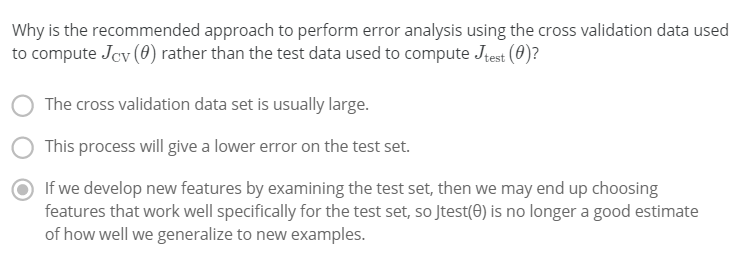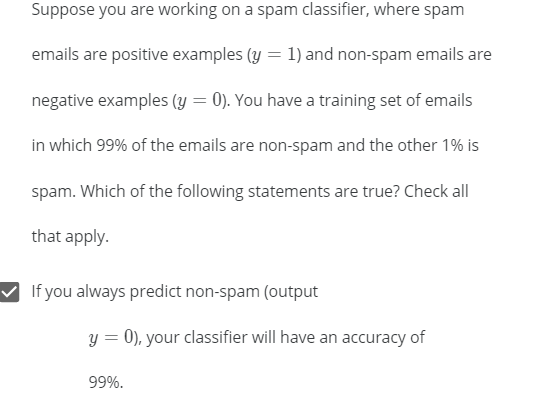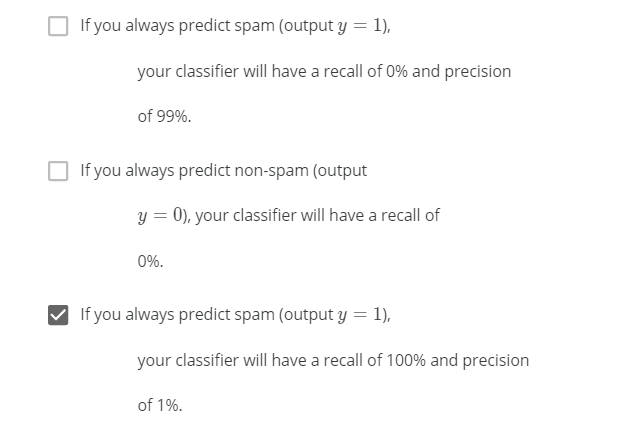# Support vector machines

Question1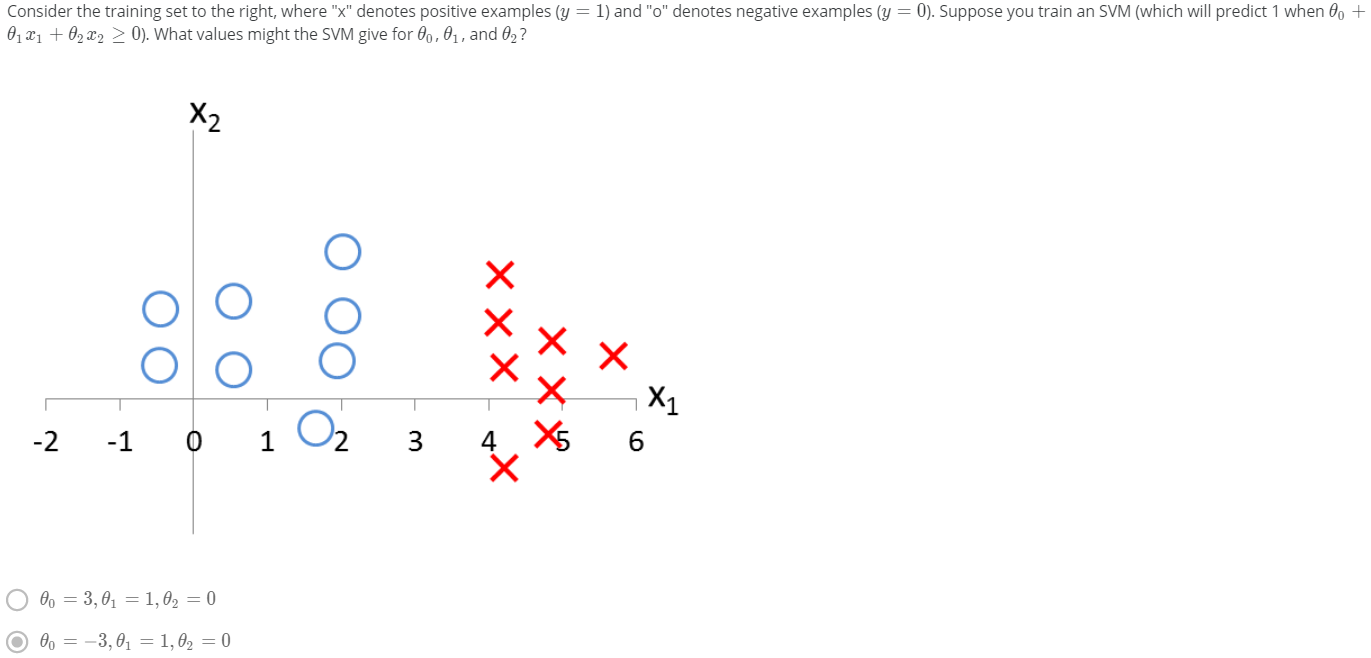Question2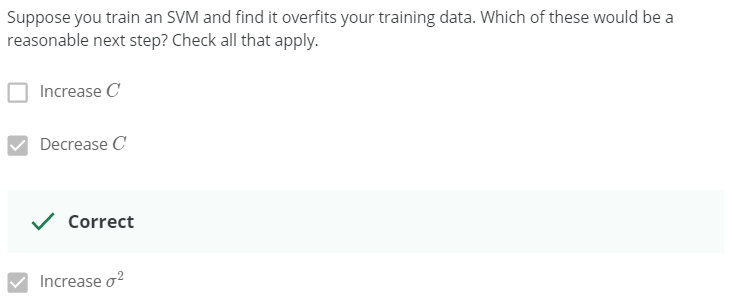Question3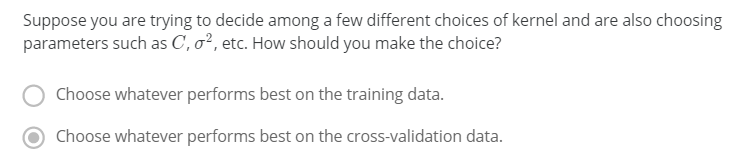Question4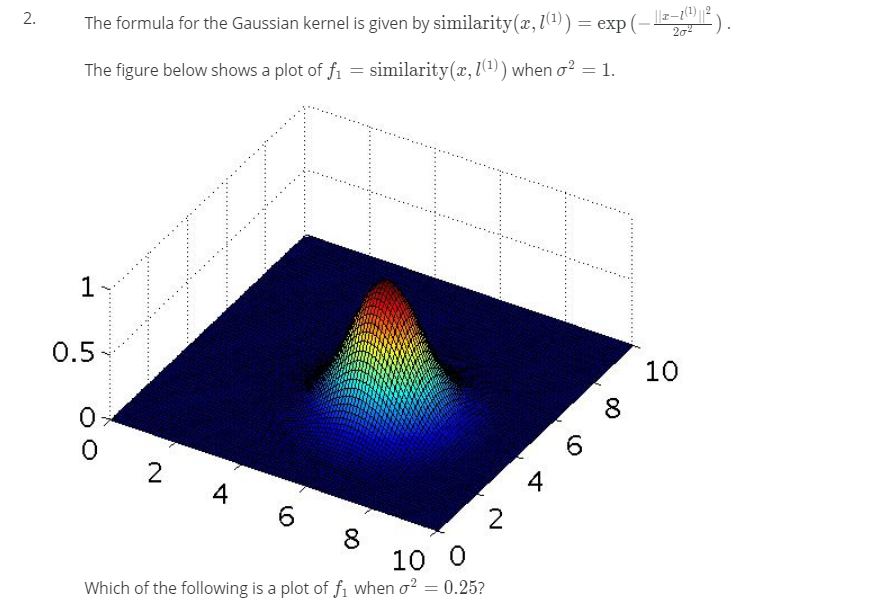Question5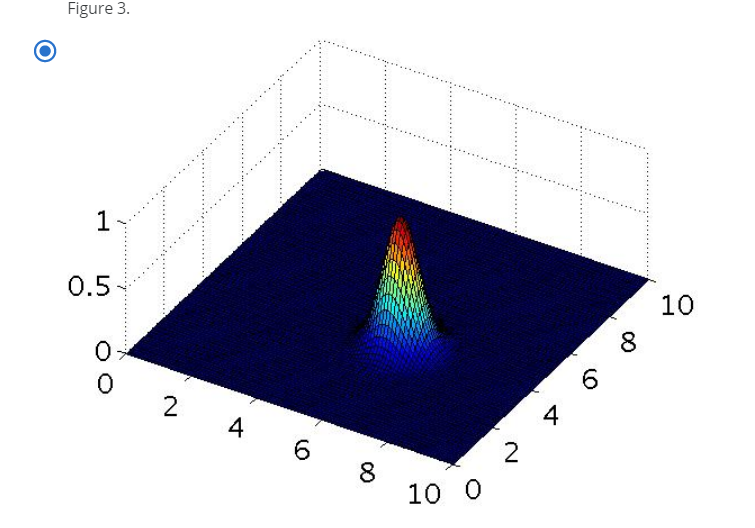Question6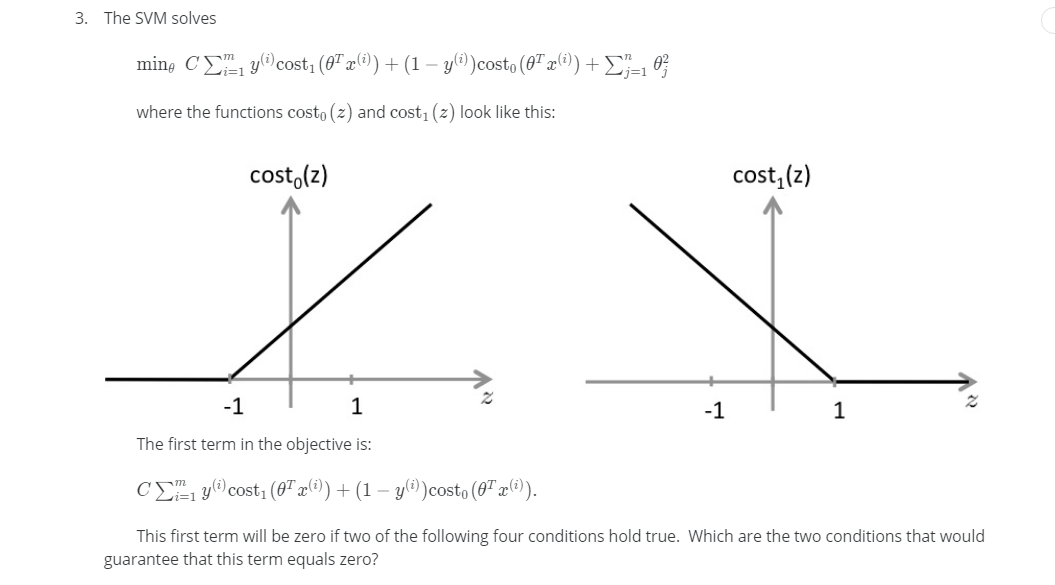Question7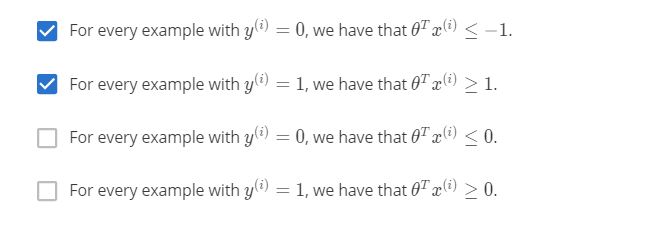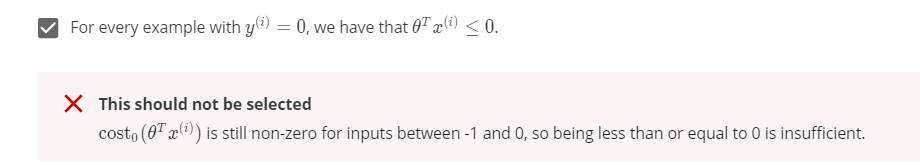Question8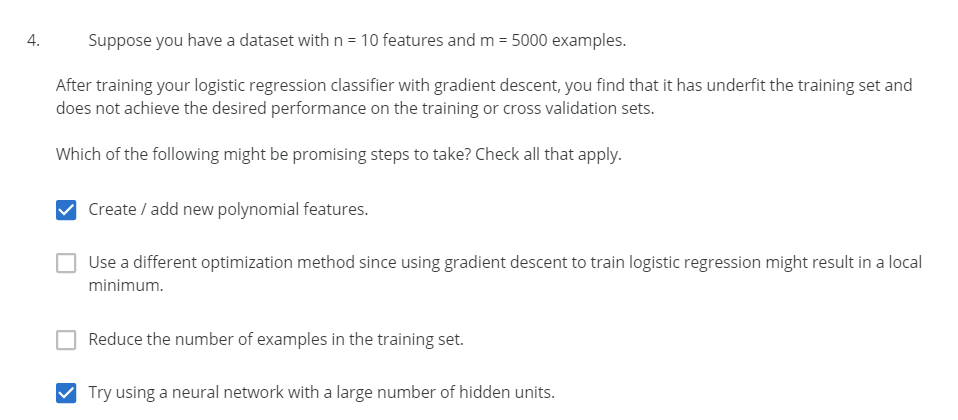# Clustering algorithm

Question1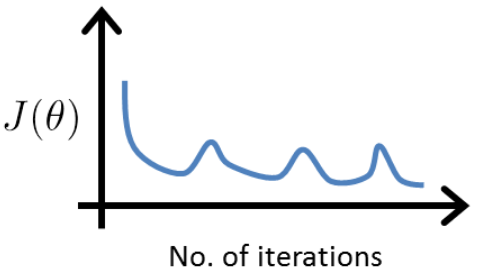It is not possible for the cost function to sometimes increase. There must be a bug in the code.

Question2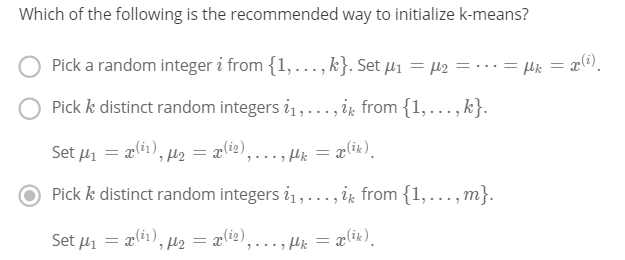Question3: Suppose you run k-means using k = 3 and k = 5. You find that the cost function J is much higher for k = 5 than for k = 3. What can you conclude?

In the run with k = 5, k-means got stuck in a bad local minimum. You should try re-running k-means with multiple random initializations.

Question4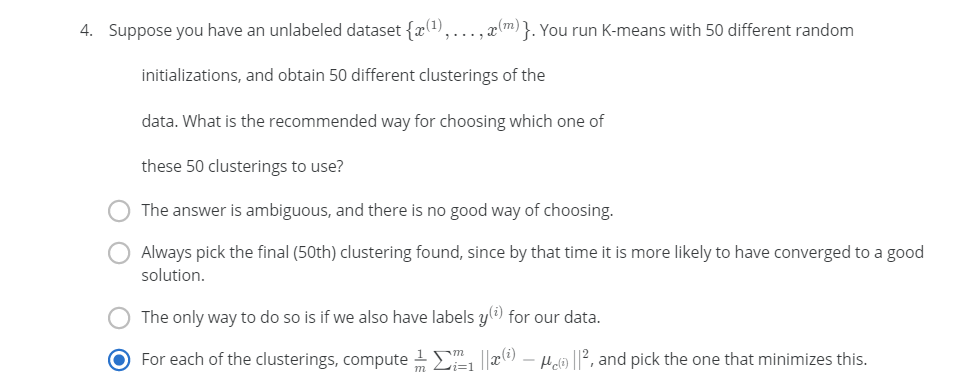Question5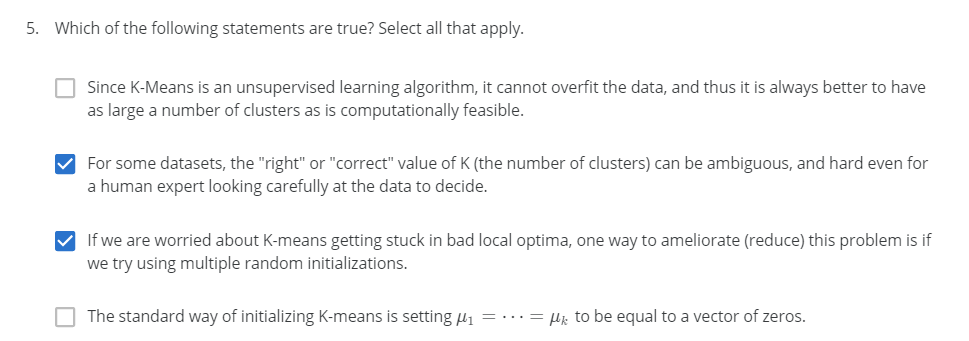# Dimensionality reduction

Question1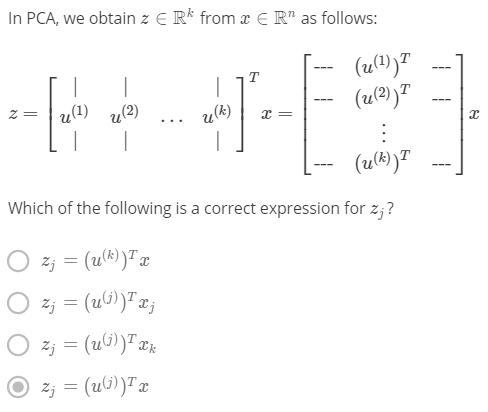Question3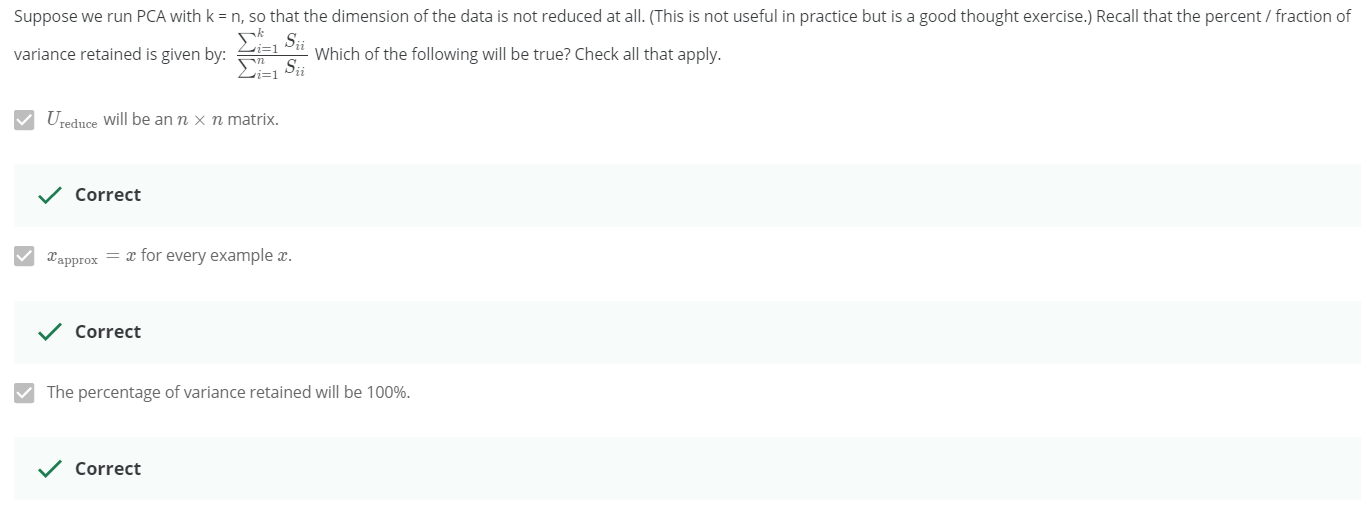Question5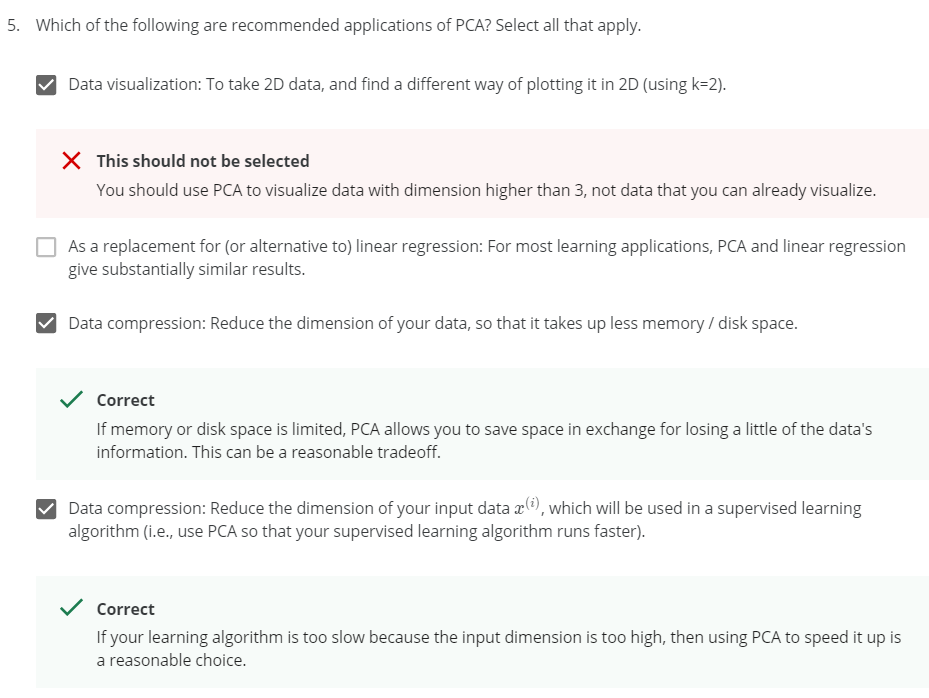# Reference

 Andrew NG, Machine learning